# Hanrui Wang¶

## Introduction¶

I'm currently a sophomore undergraduate in Electronic Information Science & Engineering(Excellent Class), School of Information Science and Engineering.

I worked in Video & Image Processing Lab affiliated to State Key Laboratory of ASIC and System, Fudan University, advised by Prof.Yibo Fan since Jan., 2016.

My research interests lie in Image processing and hardware implementation.

Now I'm doing research on hardware-oriented high-speed algorithms for Stereo Match.

The latest progress in the Stereo Match project including introduction, reference etc. can be seen in the attachment.

## Literature Investigation¶

### "HARDWARE DESIGN AND IMPLEMENTATION OF HR REAL TIME STEREO MATCHING FOR AUTOMATIC DRIVE" by Liming Wang¶

Four steps:
PART1.window size determination:
using the constant number of sample pixels as 25.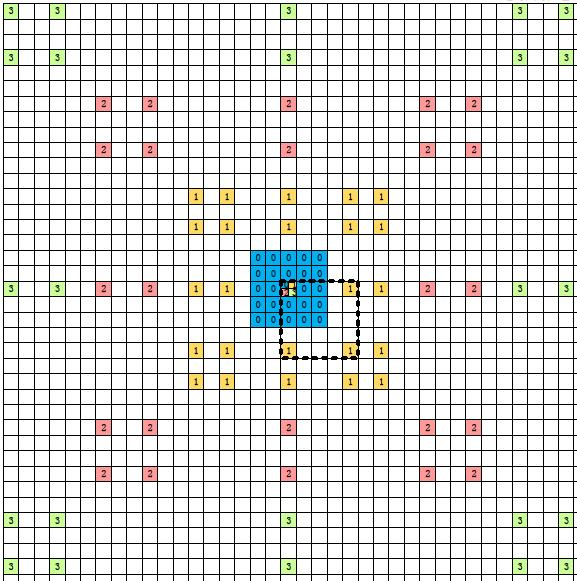using the following formula to determine the size of the window for a pixel: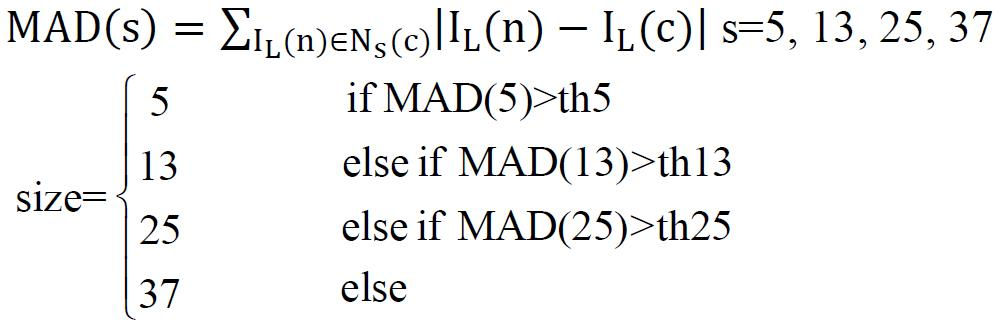PART2.cost aggregation
using the hybrid method of binary window sum of absolute value(BW-SAD) and census transform information: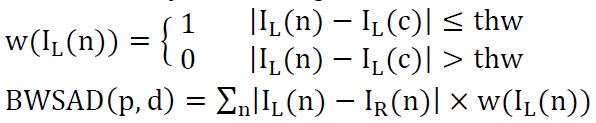Noting that only 9 out of 25 sample pixels’ accurate BW-SADs are calculated and the rest are got by linear interpolating, I’m wondering if the linear interpolating makes sense.
census: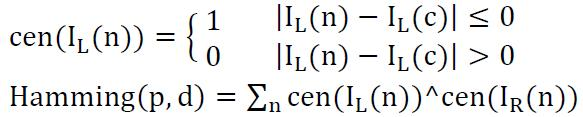hybrid method: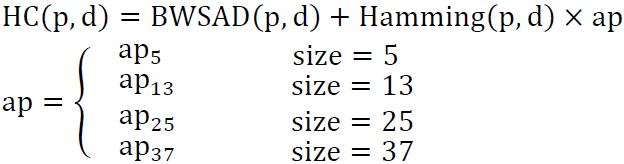Then the cost of a pixel for a certain disparity will be calculated. The disparity generating the least cost will be chosen as the final disp for one pixel.
PART3.cross check
This step aims to mark the inaccurate disp in the disp map by checking disps of the same pixels in two disp map.
The following formula is the specific method.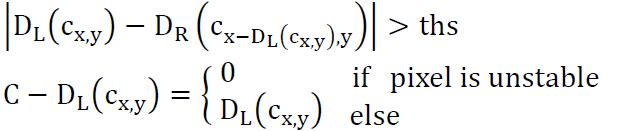The biggest leak of this method is that at the time we use the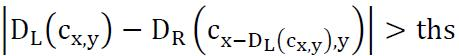formula to find the relevant pixels in the two picture, the D is not accurate yet, so chance are that the two pixels are actually irrelevant.
4.region growth
The core of this part is to fill the unstable pixels, which have been already marked in part 3, with the disp of the pixels in an identical object using the following formula.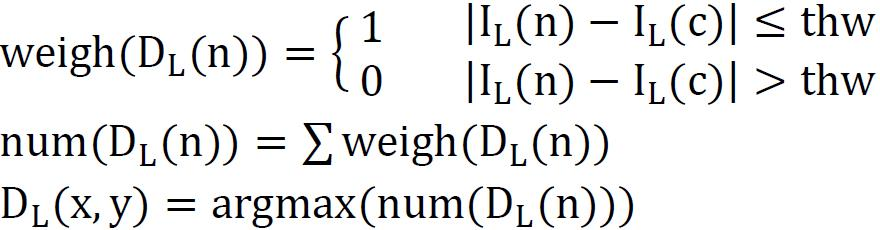What’s more, to avoid mistakes in very large low texture regions, a threshold to limit the replacement is used.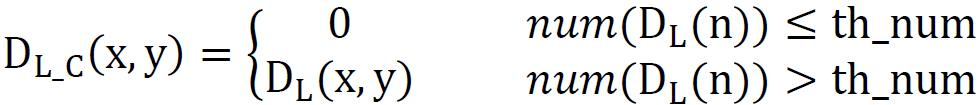### "Trinocular Adaptive Window Size Disparity Estimation Algorithm and Its Real-Time Hardware" by A.Akin¶

This paper is the improved edition of the paper” Dynamically adaptive real-time disparity estimation hardware using iterative refinement”, the methods in the two papers are similar somehow.

The biggest difference does not lie in the use of three or two pictures when getting the initial disp map but the method to determine whether the d of a pixel is accurate or not.

In this paper, to get the initial disp for a certain pixel based on the center picture, the cost of the center-left pair and center-right pair for a certain d is calculated simultaneously and the minimum and second minimum cost as well as the corresponding d are recorded as (c1,d1), (c2,d2). What should be underlined is that (c1,d1) and (c2,d2) belong to the overall matching cost computation obtained from any of the stereo pairs, which means they are not necessarily from the different pairs. What’s more, it inspires me that this methodology can also be used in the two-camera frame.

Then, a bool ambiguity is identified for that certain pixel when two low-value costs are similar while corresponding d are of significant differences.

When the ambiguity is 0, the confidence is 1 which is used in the following statement.

The specific evaluation criteria given by the author is presented as the following formula: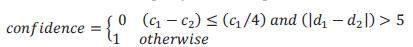In terms of the use of the confidence parameter,+* the confident disparity is used in the following refinement step and unconfident disparity values are disregarded while determining the most frequent disparity value.*+

Using the confidence metric during the refinement process eliminates a significant amount of incorrect propagations, especially within low-textured regions.

### "Iterative Disparity Voting Based Stereo Matching Algorithm and Its Hardware Implementation" by Zhi Hu¶

The algorithm proposed by Zhi Hu consists of 3 main part:Cost computation and correspondence matching, Iterative weighed disparity voting and occlusion detection and filling.

There are a lot of differences between Hu’s algorithm and Wang liming’s which will be discussed in the following statement.

Part 1 Cost computation and correspondence matching

In this paper, the window is fixed and rather small, only 3*3. Comparing to the Akin’s and Wang’s method in which much bigger adaptive windows are used,+* the small window seems to work well too, according to the result presented in the paper.*+ One the other hand, using adaptive window may improve the result.

What’s more, the+* cost aggregation method is also different*+ as the following formula: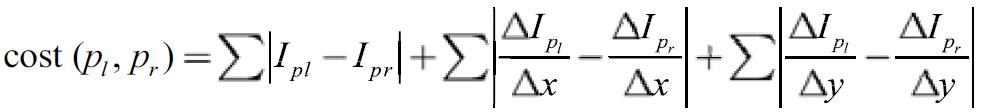Part 2 Iterative weighed disparity voting(Refinement)
In this part, the author uses many novel methods which prove to be significantly beneficial to the result.

Two mode are using in the weighted disparity voting(here the meaning of+* the term” disparity voting” is actually a part of refinement*+ which is different from that in Akin’s or Wang’s paper), one is vertical voting, the other is horizontal voting.

Vertical voting use the 21 neighbor pixels in the same column with the processing pixel as the samples and a binary parameter is also used to determine if the sample pixel is in the identical object with the processing pixel. Writing-back is used here. What’s important is that the weight of each sample pixel is quite different with the Akin’s paper as the following formula shows: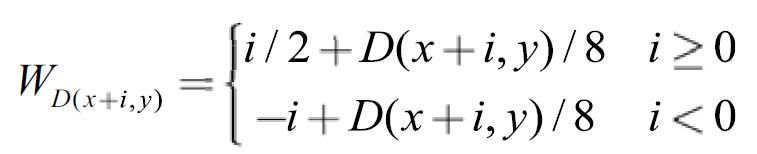The weight of the remote pixels above are larger as well as the pixels below are smaller in consideration of that the bad pixels have the inclination to agglomerate rather than scatter around evenly and the pixels above have already been refined.
And the adding of the factor D(x+i,y)/8 is beneficial for the larger disps to get bigger weight in consideration of that, empirically, the bad pixels often have quite low disps.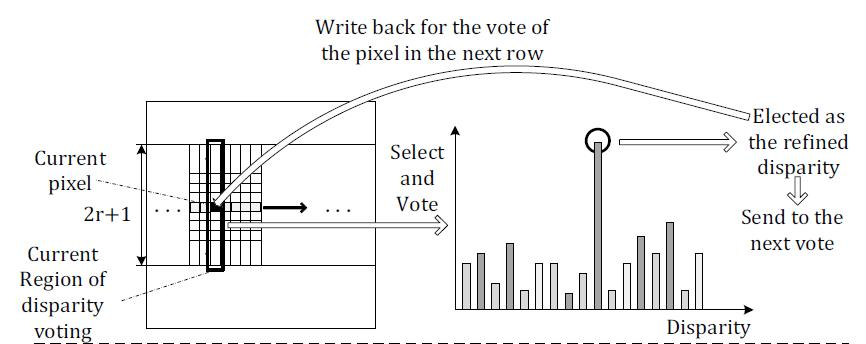Horizontal voting is much more easier than the vertical one as the weight is identically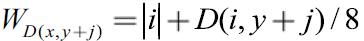and the writing-back is not used.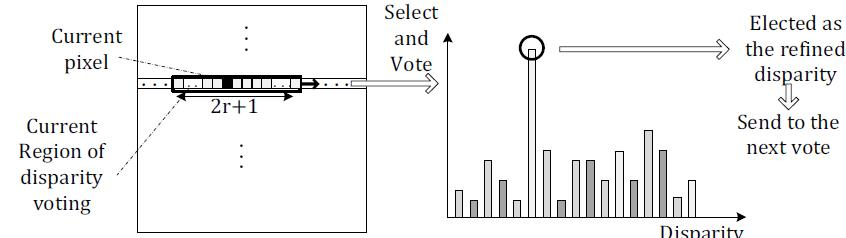Part 3 occlusion detection and filling
After two disp maps based on two images being generated, the non-occluded pixels is labeled by the formula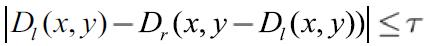which is the same as that in Wang’s paper.

However the filling method is different: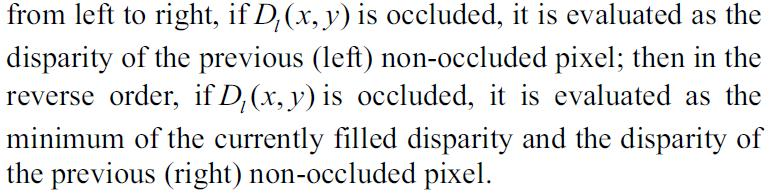The author doesn’t explain the reason and I haven’t figured out the meaning of that yet.